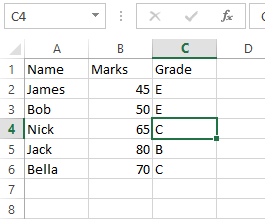# Switch Statement

To execute a group of statements depending upon the value of an Expression, then we use the Switch Case. Here, each value is called a Case, and the variable is being switched ON based on each case. Else statement case is executed if the test expression doesn’t match with any of the Case specified by the user.

Case Else is an optional statement within the select Case. However, it is a good programming practice always to have a Case Else statement.

### Syntax:

Flow diagram### Example

Let’s find the type of integer with the help of a function.

After that executes the above code, and you will get the output such as:

`The number is the least composite number   `

Let’s see some more example of the switch statement, such as

For example, you want to create a custom function (UDF) using the switch statement.

It can be used just like any regular worksheet function, and it will return the grade of the students.

The code for creating the custom formula as follows:

Now, you can use the function GetGrade in the worksheet as shown in the below screenshot, such as:Next TopicVBA Loops